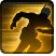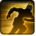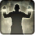# Geladener SturmConditions

## Used by

Used by
• apc.companion.weapon_set.dual_blaster.tank

## Related effects, buffs and debuffs

Please click on an effect below to view its details.

• [0s] [not displayed]Effect #1

 Slot: Debuff Duration: 0 Sek. Tick rate: does not tick # occurrences: 0
• On Apply

Only when the following conditions are met:

• If CASTER is positioned not more than 4m to melee distance of TARGET

Perform the following actions:

• Add effect #2 to TARGET from TARGET
• On Apply

Only when the following conditions are met:

• If previous subeffect did not meet all conditions

Perform the following actions:

• Add effect #3 to TARGET from TARGET
• [0s] [not displayed]Effect #2

 Slot: Debuff Duration: 0 Sek. Tick rate: does not tick # occurrences: 0 Conditions: Can only by called by other effects
• On Apply

Only when the following conditions are met:

• If TARGET does not have 1 or more effects with the tag 13954922537604821686

Perform the following actions:

• Play appearance epp . jedi_knight . force_leap . instant_no_charge, dependent on calling effect
• Weapon Damage
- Is Special Ability = (bool) true
- Ignore Dual Wield Modifier = (bool) true
- Unknown (609) = (bool) false
- Flurry Blows Min = (int) 1
- Flurry Blows Max = (int) 1
- Level Cap = (int) 0
- Threat Percent = (float) 0.5
- Standard Health Percent Max = (float) 0.164
- Standard Health Percent Min = (float) 0.164
- Amount Modifier Fixed Max = (float) 0
- Amount Modifier Fixed Min = (float) 0
- Amount Modifier Percent = (float) 0.09
- Coefficient = (float) 1.64
- Health Steal Percentage = (float) 0.25
- Slots = Int(2)
• Weapon Damage
- Is Special Ability = (bool) true
- Ignore Dual Wield Modifier = (bool) false
- Unknown (609) = (bool) false
- Flurry Blows Min = (int) 1
- Flurry Blows Max = (int) 1
- Level Cap = (int) 0
- Threat Percent = (float) 0.5
- Standard Health Percent Max = (float) 0
- Standard Health Percent Min = (float) 0
- Amount Modifier Fixed Max = (float) 0
- Amount Modifier Fixed Min = (float) 0
- Amount Modifier Percent = (float) 0.09
- Coefficient = (float) 0
- Health Steal Percentage = (float) 0.25
- Slots = Int(4)
• On Apply

Only when the following conditions are met:

• If TARGET has an effect with the tag 13954922537604821686

Perform the following actions:

• Play appearance epp . jedi_knight . force_leap . instant_no_charge, dependent on calling effect
• Weapon Damage
- Is Special Ability = (bool) true
- Ignore Dual Wield Modifier = (bool) true
- Unknown (609) = (bool) false
- Flurry Blows Min = (int) 1
- Flurry Blows Max = (int) 1
- Level Cap = (int) 0
- Threat Percent = (float) 0.5
- Standard Health Percent Max = (float) 0.164
- Standard Health Percent Min = (float) 0.164
- Amount Modifier Fixed Max = (float) 0
- Amount Modifier Fixed Min = (float) 0
- Amount Modifier Percent = (float) 0.09
- Coefficient = (float) 1.64
- Health Steal Percentage = (float) 0.5
- Slots = Int(2)
• Weapon Damage
- Is Special Ability = (bool) true
- Ignore Dual Wield Modifier = (bool) false
- Unknown (609) = (bool) false
- Flurry Blows Min = (int) 1
- Flurry Blows Max = (int) 1
- Level Cap = (int) 0
- Threat Percent = (float) 0.5
- Standard Health Percent Max = (float) 0
- Standard Health Percent Min = (float) 0
- Amount Modifier Fixed Max = (float) 0
- Amount Modifier Fixed Min = (float) 0
- Amount Modifier Percent = (float) 0.09
- Coefficient = (float) 0
- Health Steal Percentage = (float) 0.5
- Slots = Int(4)
• [0s] [not displayed]Effect #3

 Slot: Debuff Duration: 0 Sek. Tick rate: does not tick # occurrences: 0 Conditions: Can only by called by other effects
• On Apply

Only when the following conditions are met:

• If TARGET does not have 1 or more effects with the tag 13954922537604821686

Perform the following actions:

• Teleport To Target
• Add effect #4 to TARGET from TARGET, Combat Math Only
• Play appearance epp . agent . teleport . instant_cast, dependent on calling effect
• Weapon Damage
- Is Special Ability = (bool) true
- Ignore Dual Wield Modifier = (bool) true
- Unknown (609) = (bool) false
- Flurry Blows Min = (int) 1
- Flurry Blows Max = (int) 1
- Level Cap = (int) 0
- Threat Percent = (float) 0.5
- Standard Health Percent Max = (float) 0.164
- Standard Health Percent Min = (float) 0.164
- Amount Modifier Fixed Max = (float) 0
- Amount Modifier Fixed Min = (float) 0
- Amount Modifier Percent = (float) 0.09
- Coefficient = (float) 1.64
- Health Steal Percentage = (float) 0.25
- Slots = Int(2)
• Weapon Damage
- Is Special Ability = (bool) true
- Ignore Dual Wield Modifier = (bool) false
- Unknown (609) = (bool) false
- Flurry Blows Min = (int) 1
- Flurry Blows Max = (int) 1
- Level Cap = (int) 0
- Threat Percent = (float) 0.5
- Standard Health Percent Max = (float) 0
- Standard Health Percent Min = (float) 0
- Amount Modifier Fixed Max = (float) 0
- Amount Modifier Fixed Min = (float) 0
- Amount Modifier Percent = (float) 0.09
- Coefficient = (float) 0
- Health Steal Percentage = (float) 0.25
- Slots = Int(4)
• On Apply

Only when the following conditions are met:

• If TARGET has an effect with the tag 13954922537604821686

Perform the following actions:

• Teleport To Target
• Add effect #4 to TARGET from TARGET, Combat Math Only
• Play appearance epp . agent . teleport . instant_cast, dependent on calling effect
• Weapon Damage
- Is Special Ability = (bool) true
- Ignore Dual Wield Modifier = (bool) true
- Unknown (609) = (bool) false
- Flurry Blows Min = (int) 1
- Flurry Blows Max = (int) 1
- Level Cap = (int) 0
- Threat Percent = (float) 0.5
- Standard Health Percent Max = (float) 0.164
- Standard Health Percent Min = (float) 0.164
- Amount Modifier Fixed Max = (float) 0
- Amount Modifier Fixed Min = (float) 0
- Amount Modifier Percent = (float) 0.09
- Coefficient = (float) 1.64
- Health Steal Percentage = (float) 0.5
- Slots = Int(2)
• Weapon Damage
- Is Special Ability = (bool) true
- Ignore Dual Wield Modifier = (bool) false
- Unknown (609) = (bool) false
- Flurry Blows Min = (int) 1
- Flurry Blows Max = (int) 1
- Level Cap = (int) 0
- Threat Percent = (float) 0.5
- Standard Health Percent Max = (float) 0
- Standard Health Percent Min = (float) 0
- Amount Modifier Fixed Max = (float) 0
- Amount Modifier Fixed Min = (float) 0
- Amount Modifier Percent = (float) 0.09
- Coefficient = (float) 0
- Health Steal Percentage = (float) 0.5
- Slots = Int(4)
• [2s]Gelähmt (Physisch)
Bewegungsunfähig.

 Slot: Debuff Duration: 2 Sek. Tick rate: does not tick # occurrences: 1 Tags: tag.​abl.​debuff.​root, tag.​abl.​dispel_type.​physical Conditions: Can only by called by other effects
• On Apply

Perform the following actions:

• Play appearance epp . sith_warrior . force_slow . target_debuff, dependent on calling effect
• Immobilize but allow rotation